Contents

### Context

#### Higher algebra

higher algebra

universal algebra

# Contents

## Idea

A compactification of configuration spaces of points was introduced in Axelrod-Singer 93, p. 5-6, Fulton-MacPherson 94 and an operad-structure defined on it by Getzler-Jones 94 Kontsevich 97, now called the Fulton-MacPherson operad, weakly equivalent to the little n-disk operad (Salvatore 01, Prop. 4.9), hence a model for an En-operad.

The de Rham cohomology as well as the de Rham complex itself of the Fulton-MacPherson operad it quasi-isomorphic to graph complexes via topological Feynman amplitudes (see below), in fact it is the perturbative computation of Feynman amplitudes in Chern-Simons theory that motivated the compactified configuration space, this way, in Axelrod-Singer 91, Axelrod-Singer 93. These two quasi-isomorphism serve to exhibit formality of the Fulton-MacPherson operad, and hence also formality of the little n-disk operad.

To see the nature of the Axelrod-Singer-Fulton-MacPherson compactification of configuration spaces of points, observe that the configuration space of exactly 2 points in a Cartesian space/Euclidean space $\mathbb{R}^d$ is homotopy equivalence to the quotient by Z/2 of a (d-1)-sphere

$Conf_2( \mathbb{R}^d ) \;\simeq\; S^{d-1}/(\mathbb{Z}/2)$

this being the sphere of directions of the vector pointing from one of the two points to the other. This means that the configuration space $Conf_n( \mathbb{R} )$ of $n$ points is homotopy equivalent to a quotient by the symmetric group $\Sigma(n)$ of the Cartesian product of one such (d-1)-sphere of directions for each pair of points and various open intervals encoding the relative distance between the points.

The Fulton-MacPherson compactification is the evident compactification of this quotient of Cartesian products of (d-1)-spheres with open intervals, replacing the latter by their corresponding closed intervals.

This means that a point on the boundary of the Fulton-MacPherson compactification corresponds to a would-be configuration of points where some of the points have formally vanishing distance to each other, while still remembering a relative direction to each other.

More abstractly one may sum this up succinctly by saying that the ASFM-compactification is the blowup of the fat diagonal inside the Cartesian products of the base space with itself.

In the literature these boundary configurations are often referred to in terms of “infinitesimally close points”. While there may be some formal relation to actual infinitesimal neighbourhoods in (for example) synthetic differential geometry, we point out the Axelrod-Singer-Fulton-MacPherson compactifications as defined below are plain topological spaces or manifolds with corners (in some suitable sense), not objects in a smooth topos in any of the usual senses.

## Definition

For $n, d \in \mathbb{N}$, consider first the topological space of configurations of ordered n-tuples of points in the Cartesian space/Euclidean space $\mathbb{R}^d$, hence the mapping space of injections/embeddings of topological spaces

$Emb( \{1, \cdots, n\}, \mathbb{R}^d ) \;\subset\; \left( \mathbb{R}^d \right)^n \,.$

The group of translations and of scale transformations acts canonically on $\mathbb{R}^d$ and hence diagonally on $Emb( \{1, \cdots, n\}, \mathbb{R}^d )$. The resulting quotient topological space

$Emb( \{1, \cdots, n\}, \mathbb{R}^d ) \longrightarrow Emb( \{1, \cdots, n\}, \mathbb{R}^d )/ \mathbb{R}^d \rtimes \mathbb{R}_{\gt 0}$

is clearly homotopy equivalent to the original space.

But now for $n \geq 2$ this quotient space canonically carries the structure of a topological manifold (in fact of a smooth manifold) of dimension

$dim \left( Emb\left( \{1, \cdots, n\}, \mathbb{R}^d \right)/\left( \mathbb{R}^d \rtimes \mathbb{R}_{\gt 0}\right) \right) \;=\; n \cdot d - d - 1$

while for $n \leq 1$ the quotient is the point space (of dimension 0).

Now for a pair $i \neq j \in \{1, \cdots, n\}$ of distinct indices, consider the continuous function

(1)$\array{ Emb\left( \{1, \cdots, n\}, \mathbb{R}^d \right)/\left( \mathbb{R}^d \rtimes \mathbb{R}_{\gt 0}right) \right) &\overset{\theta_{i j}}{\longrightarrow}& S^{d-1} \subset \mathbb{R}^d \\ (x_1, \cdots, x_n) &\mapsto& \frac{ x_j - x_i }{ {\vert x_j - x_i\vert} } }$

which takes each configuration to the direction of the vector between the $i$th and the $j$th point in the configuration.

Moreover, for each triple $i,j,k$ of mutually distinct indices, consider the continuous function

$\array{ Emb\left( \{1, \cdots, n\}, \mathbb{R}^d \right)/\left( \mathbb{R}^d \rtimes \mathbb{R}_{\gt 0}\right) &\overset{\delta_{i j k}}{\longrightarrow}& (0,\infty) \subset [0,\infty] \\ (x_1, \cdots, x_n) &\mapsto& \frac{ {\vert x_i - x_j\vert} }{ {\vert x_j - x_k \vert} } }$

which takes each configuration to the ratio of the distance between the $i$th and the $j$th point over that of the $j$th to the $k$th point.

Together these functions induce one total continuous function

(2)$\iota \;\colon\; Emb\left( \{1, \cdots, n\}, \mathbb{R}^d \right)/\left( \mathbb{R}^d \rtimes \mathbb{R}_{\gt 0}\right) \overset{ (\theta_{i j}, \delta_{i j k}) }{\hookrightarrow} \left( S^{d-1} \right)^{ n(n-1) } \times [0,\infty]^{ \left( n(n-1)(n-2) \right) }$

from the ordered configuration space into the Cartesian product of all these (d-1)-spheres and intervals.

A moment of reflection shows that every ordered configuration modulo translation and rescaling, hence every point on the left, may be uniquely reconstructed from its direction vectors and distance ratios, hence from a point on the right, hence that this function is a homeomorphism onto its image (Sinha 03, lemma 3.18).

###### Definition

(Fulton-MacPherson compactification)

The Fultan-MacPherson compactification of the configuration space of ordered n-tuples of points in the Cartesian space/Euclidean space $\mathbb{R}^d$ is the topological closure of the image of (2):

$FM_n(\mathbb{R}^d) \;\coloneqq\; \overline{ \iota\left( Emb\left( \{1, \cdots, n\}, \mathbb{R}^d \right)/\left( \mathbb{R}^d \rtimes \mathbb{R}_{\gt 0}\right) \right) }$

The symmetric group $\Sigma(n)$ still canonically acts on $FM_n(\mathbb{R}^d)$ and hence the FM-compactification of the actual configuration space of points $Conf_n(\mathbb{R}^d)$ is the quotient space

$\overline{Conf_n(\mathbb{R}^d)} \;=\; FM_n(\mathbb{R}^d)/\Sigma(n) \,.$

## Pictorial notation

By the above, the points on the boundary of a Fulton-MacPherson compactification of configuration spaces of points (Def. ) correspond to configurations that involve triples of points in space $x,y,z$, such that the distance between two of them is “vanishing in ratio to the distance of both to the third”.

Hence where a configuration of two points in space looks like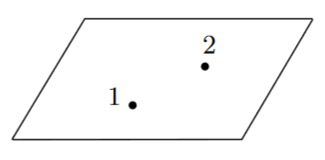a configuration of such a boundary case of three points may be visualized as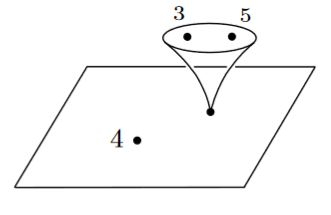where the “funnel” might be thought of as an infinite magnifying glass held to the location of the second point and revealing that it really consists of two points of vanishing distance to each other, relative to their joint distance to the remaining point.

In this fashion, for instance a boundary point in the Fulton-MacPherson compactification depicted as follows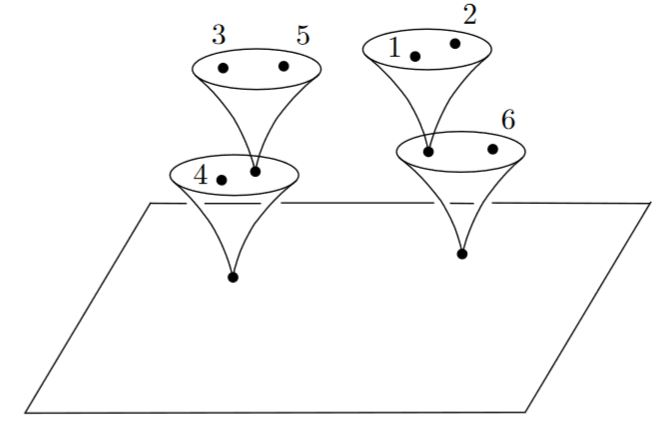would consist of

• points 3, 4 and 5 being arbitrarily close to each other with respect to their distance to points 1, 2 and 6

• points 3 and 5 also being arbitrarily close to each other with respect to their joint distance to 4

• points 1, 2 and 6 being arbitrarily close to each other in comparison to their joint distances to 3, 4 and 5

• points 1 and 2 also being arbitrarily close to each other with respect to their joint distance to 6.

graphics grabbed from Lambrechts-Volic 14, Figures 3 and 4

This pictorial notation was introduced in Sinha 03. It immediately suggests the correct operad-structure on the Fulton-MacPherson compactifications.

## Properties

### Relation to the little $n$-disk operad

###### Proposition

The Fulton-MacPherson operad $FM_\bullet\left( \mathbb{R}^d\right)$ is weakly equivalent in the model structure on operads with respect to the classical model structure on topological spaces, to the little d-disk operad.

(Salvatore 01, Prop. 4.9, summarized as Lambrechts-Volic 14, Prop. 5.6)

### de Rham cohomology

We discuss the de Rham complex $\Omega^\bullet(FM_n(\mathbb{R}^d))$ and the de Rham cohomology $H^\bullet_{dR}( FM_n(\mathbb{R}^d) )$ of Fulton-MacPherson compactifications.

###### Definition

Let $d \in \mathbb{N}$ (dimension of Euclidean space), and $n \in \mathbb{N}$ (number of points) and consider the Fulton-MacPherson compactification $FM_n(\mathbb{R}^d)$ from Def. .

Let

$dvol_{\left(S^{d-1}\right)} \;\in\; \Omega^\bullet\left( S^{d-1} \right)$

be the standard volume form on the standard d-sphere.

For $i \neq j \in \{1, \cdots, n\}$ two distinct point labels, write

$g_{i j} \;\coloneqq\; \left(\theta_{i j}\right)^\ast\left( dvol_{\left( S^{d-1}\right)}\right) \;\in\; \Omega^\bullet\left( FM_n\left(\mathbb{R}^d\right) \right)$

be the pullback of differential forms of this standard volume form along the projection $\theta_{i j} \;\colon\; FM_n\left( \mathbb{R}^{d}\right) \longrightarrow S^{d-1}$ from (1).

###### Proposition

Let $d \in \mathbb{N}$ (dimension of Euclidean space), and $n \in \mathbb{N}$ (number of points) and consider the Fulton-MacPherson compactification $FM_n(\mathbb{R}^d)$ from Def. .

The de Rham cohomology of $FM_n\left( \mathbb{R}^d\right)$ is, as a graded-commutative algebras, isomorphic to the quotient algebra of the free graded commutative algebra generated by the classes of the forms $g_{i j}$ from Def.

$H^\bullet_{dR}\left( FM_n\left( \mathbb{R}^d \right) \right) \;\simeq\; \wedge_{\mathbb{R}}^\bullet \left\langle \left[g_{i j}\right] \;\vert\; i \neq j \in \{1, \cdots, n\} \right\rangle / \sim$

by the following three relations (for all distinct $i,j,k \in \{1,\cdots, n\}$):

1. $\left[g_{i j}\right] \;\sim\; (-1)^d \left[g_{j i}\right]$;

2. $\left[g_{i j}\right] \wedge \left[g_{i j}\right] \;\sim\; 0$;

3. $\left[g_{i j}\right] \wedge \left[ g_{j k} \right] + \left[g_{j k}\right] \wedge \left[ g_{k i} \right] + \left[g_{k i}\right] \wedge \left[ g_{i j} \right] \;\sim\; 0$ $\;\;\;\;$(“3-term relation”)

This is due to (Arnold 69, Cohen 73).

###### Remark

(real cohomology of the FM-compactification in terms of graph cohomology)

In the graph complex-model for the rational homotopy type of the ordered configuration space of points $Conf_n\big( \mathbb{R}^D\big)$ the three relations in Prop. are incarnated as follows:

1. a graph changes sign when one of its edges is reversed (this Def.)

2. a graph with parallel edges is a vanishing graph (this Def.)

3. the graph coboundary of a single trivalent internal vertex (this Example).

### Relation to Graph complexes and Formality theorem

We have that the Fulton-MacPherson operad is formal in the sense that for each of its component manifolds $FM_n\left( \mathbb{R}^d\right)$ (Def. ) there is a zig-zag of quasi-isomorphisms between their de Rham cohomology and their de Rham complex, and such that these morphisms are compatible with the induced cooperad-structure on both sides.

Concretely, the zig-zags may be taken to consist of one span of quasi-isomorphisms out of a suitable graph complex to the de Rham cohomology/de Rham complex of the Fulton-MacPherson operad.

Here the morphism from the graph complex to the de Rham complex of the Fulton-MacPherson operad regards the latter as the compactification of a configuration space of points, regards functions/differential forms on configuration spaces of points as n-point functions of a topological quantum field theory, regards suitable graphs as Feynman diagrams and proceeds by sending each such graph/Feynman diagram to a corresponding Feynman amplitude.

This idea of a proof was sketched in Kontsevich 99, a full account is due to Lambrechts-Volic 14.

###### Definition

(the graph complex)

For $n\in \mathbb{N}$, write

$Graphs_n\left( \mathbb{R}^d \right) \;\in\; dgcAlg_{\mathbb{R}}$

for the graph complex of “Feynman graphs” with $n$ external vertices, regarded with its structure of a differential graded-commutative algebra over the real numbers.

###### Proposition
$\overline{I} \;\colon\; Graphs_n\left( \mathbb{R}^d\right) \overset{ \phantom{AA}\simeq \phantom{AA} }{\longrightarrow} H^\bullet_{dR}\left( FM_n\left( \mathbb{R}^d \right) \right)$

from the graph complex dgc-algebra (Def. ) to the de Rham cohomology (Prop. ) of the Fulton-MacPherson compactification (Def. ) given on generators by

$\overline{I}\left( \Gamma \right) \;=\; \left\{ \array{ \left[g_{i j}\right] &\vert& \Gamma \, {\text{is diagram without internal vertices} \atop \text{and single edge between external vertices}\, i \, \text{and}\, j} \\ 0 &\vert& \Gamma \, \text{has internal vertices} } \right.$

(where $g_{i j} \in \Omega^\bullet\left( FM_n\left( \mathbb{R}^d\right) \right)$ are the differential forms from Def. )

extends to a homomorphism of dgc-algebras which is a quasi-isomorphism.

###### Example

(the “3-term relation”)

In the graph complex the differential of the graph as shown on the left below (the vertices on the horizontal line are the external vertices, that above the line is internal) is a linear combination as shown on the right:

$\phantom{AAA}\array{ \partial \\ \phantom{A} \\ \phantom{a}}$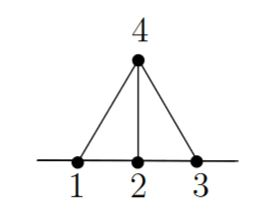$\phantom{A}\array{ = \\ \phantom{A} \\ \phantom{a} }\phantom{A}$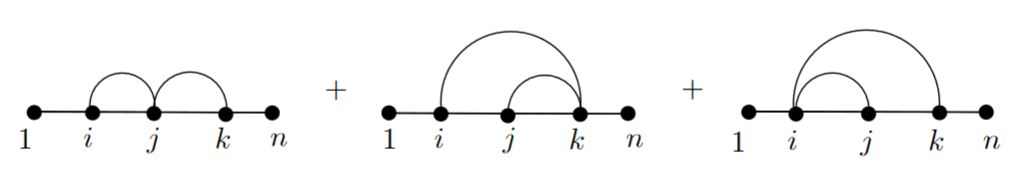graphics grabbed from Lambrechts-Volic 14, Figure 1 & Figure 2

Under the above quasi-isomorphism (Prop. ) from the graph complex to the de Rham complex on the Fulton-MacPherson compactification of a configuration space of points given by sending each graph to its Chern-Simons Feynman amplitude on compactified configuration spaces of points (Remark ) this relation becomes the “3-term relation” from Prop.

$\left[g_{i j}\right] \wedge \left[ g_{j k} \right] + \left[g_{j k}\right] \wedge \left[ g_{k i} \right] + \left[g_{k i}\right] \wedge \left[ g_{i j} \right] \;\sim\; 0$

satisfied by the Chern-Simons propagator form

$\left( g_{i j} \;\in\; \Omega^2\left( FM_n(\mathbb{R}^d) \right) \right) \,.$

Now we consider the analogous but richer quasi-isomorphism from the graph complex not just to the de Rham cohomology of the compactified configuration space, but its full de Rham complex.

###### Definition

(fiber integration over internal vertices)

For $\Gamma \in Graphs_n\left( \mathbb{R}^d \right)$ an element in the graph complex (Def. ) with $k$ internal vertices, write

$\array{ FM_{n + k}\left( \mathbb{R}^d \right) \\ \Big\downarrow {}^{\mathrlap{\pi_n^{n + k}}} \\ FM_{n}\left( \mathbb{R}^d\right) }$

for the evident continuous function between the Fulton-MacPherson compactifications of ordered configuration spaces of points (Def. ) obtained by forgetting the $k$ “internal” of the total of $n + k$ points in a configuration.

This canonically carries the structure of a fiber bundle of semi-algebraic manifolds and hence induces a fiber- integration of differential forms from minimal to partially algebraic differential forms:

$\underset{ \pi_n^{n + k} }{\int} \;\colon\; \Omega^\bullet_{min} \left( FM_{n + k}\left( \mathbb{R}^d \right) \right) \longrightarrow \Omega^{\bullet - d \cdot k}_{PA} \left( FM_n\left( \mathbb{R}^d \right) \right)$
###### Proposition

(quasi-isomorphism between graph complex and de Rham complex of Fulton-MacPherson compactification)

Consider the linear function

$\overline{I} \;\colon\; Graphs_n\left( \mathbb{R}^d\right) \overset{ \phantom{AA}\simeq \phantom{AA} }{\longrightarrow} \Omega^\bullet_{PA}\left( FM_n\left( \mathbb{R}^d \right) \right)$

from the graph complex dgc-algebra (Def. ) to the semialgebraic de Rham complex (Def. ) of the Fulton-MacPherson compactification (Def. ) given on graphs

$\Gamma \;\in\; Graphs_{n}\left( \mathbb{R}^d\right)$

with

$k \coloneqq int(\Gamma)$

internal vertices by the integral transform of the canonical volume form through the span

$\array{ && FM_{n + k}\left( \mathbb{R}^d\right) \\ & {}^{ \mathllap{ \left( \theta_{a b} \vert a \neq b\in \{1, \cdots, n + k\} \right) } }\swarrow && \searrow^{ \mathrlap{ \pi_n^{n + k} } } \\ \left( S^{d-1} \right)^{n + k} && && FM_n\left( \mathbb{R}^d \right) }$

where

1. the function on the left, to the Cartesian product of one (d-1)-spheres for each pair of distinct vertices, is given by the functions $\theta_{a b}$ from (1)

2. the function on the right is the canonical forgetful projection from Def. ,

hence which sends

$\Gamma \;\mapsto\; \underset{ \pi_{n}^{n + int(\Gamma)} }{\int} \underset{ (a \to b) \in \Gamma }{\wedge} g_{a b}$

to the fiber integration over internal vertices (Def. ) of the wedge product of the differential forms

$g_{a b} \coloneqq \theta_{a b}^\ast dvol_{\left(S^{d-1}\right)}$

(Def. ), one for each edge in the graph from vertex $a$ to vertex $b$.

This function constitutes a homomorphism of dgc-algebras which is a quasi-isomorphism.

That this is a dgc-algebra-homomorphism compatible with the cooperad-structure is proven in Lambrechts-Volic 14, that it is a quasi-isomorphism is proven in Lambrechts-Volic 14, section 10.

###### Remark

(perturbative Chern-Simons theory )

The differential forms $g_{i j}$ from Def. may be understood as being the Feynman propagator of perturbative higher Chern-Simons theory regarded equivalently in its incarnation as a Feynman amplitude on compactified configuration spaces of points. See at Chern-Simons propagator for more on this.

From this perspective, the quasi-isomorphism in Prop. sends each graph, regarded as a Feynman diagram for higher Chern-Simons theory, to its correspomnding Feynman amplitude, regarded in its incarnation as a Feynman amplitude on compactified configuration spaces of points.

That this should be the case was originally suggested in Kontsevich 94.

The compactification of configuration spaces of points was first considered for two points in

and then more generally in

based on the general method of resolution of singularities due to

• Heisuke Hironaka, Resolution of Singularities of an Algebraic Variety Over a Field of Characteristic Zero: I, Annals of Mathematics Second Series, Vol. 79, No. 1 (Jan., 1964), pp. 109-203 (95 pages) (jstor:1970486)

More general compactifications of spaces of subspace arrangements are due to

• Eva Maria Feichner, De Concini–Procesi Wonderful Arrangement Models: A Discrete Geometer’s Point of View, Math. Sci. Res. Inst. Publ 52, 2005 (pdf)

The operad-structure on Axelrod-Singer Fulton-MacPherson compactifications of configuration spaces of points was considered in

and alternatively in

which was corrected in

• Giovanni Gaiffi, Models for real subspace arrangements and stratified manifolds, Int. Math. Res. Not. 12:627-656, 2003 (pdf)

and developed in detail in

• Dev Sinha, Manifold theoretic compactifications of configuration spaces, Selecta Math. (N.S.) 10(3):391-428, 2004 (arXiv:math/0306385)

which also shows the equivalence to Fulton-MacPherson 94.

Review includes

The de Rham cohomology was determined in

The equivalence to the little n-disk operad was established in

• Paolo Salvatore, Configuration spaces with summable labels, Cohomological methods in homotopy theory. Birkhäuser, Basel, 2001. 375-395.

The interpretation of the quasi-isomorphism establishing formality of the little n-disk operad as sending edges to Feynman propagators of some topological quantum field theory such as Chern-Simons theory is due to

which is recalled and recast in somewhat more generality in

That this should exhibit a graph complex model for the cohomology of compactified configuration spaces was suggested in

• Maxim Kontsevich, pages 11-12 of Feynman diagrams and low-dimensional topology, First European Congress of Mathematics, 1992, Paris, vol. II, Progress in Mathematics 120, Birkhäuser (1994), 97–121 (pdf)

and spelled out in more detail in

with a careful proof being layed out in